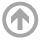Mathematics Department

# Combinatorics, Algebra, & Topology Seminar

## Spring 2021

• Mar
04
• Matrix methods for harmonic graph morphisms with applications to graph products
Caroline Melles
USNA
Time: 12:00 PM

#### View Abstract

Harmonic morphisms between graphs are analogous to holomorphic maps between Riemann surfaces. Non-constant harmonic morphisms are surjective and behave well with respect to critical groups (sandpile groups) of graphs. In particular, if there is a non-constant harmonic morphism from a graph G to a graph H, then the number of spanning trees of H divides the number of spanning trees of G. For example, there is a nontrivial harmonic morphism from the cycle graph Cn to the cycle graph Cm if and only if m divides n. We give a matrix criterion for harmonic graph morphisms in terms of the adjacency matrices of the graphs. This criterion allows us to translate combinatorial questions about harmonic morphisms to linear algebra questions. We illustrate its use by showing that certain maps associated to standard graph products are harmonic. We examine maps of NEPS (noncomplete extended p-sum) graphs, such as tensor products and Cartesian products, as well as maps of lexicographic products. Our results on harmonic maps of products provide a large number of examples of harmonic morphisms. The talk represents joint work with David Joyner.

#### ARCHIVES